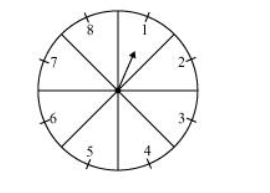# A game of chance consists of spinning an arrow which comes to rest pointing at one of the numbers

Question:

A game of chance consists of spinning an arrow which comes to rest pointing at one of the numbers 1, 2, 3, 4, 5, 6, 7, 8 (see the given figure), and these are equally likely outcomes. What is the probability that it will point at

(i) 8?

(ii) an odd number?

(iii) a number greater than 2?

(iv) a number less than 9?Solution:

Total number of possible outcomes = 8

(i) Probability of getting $8=\frac{\text { Number of favourable outcomes }}{\text { Number of total possible outcomes }}=\frac{1}{8}$

(ii) Total number of odd numbers on spinner $=4$

Probability of getting an odd number $=\frac{\text { Number of favourable outcomes }}{\text { Number of total possible outcomes }}$

$=\frac{4}{8}=\frac{1}{2}$

(iii) The numbers greater than 2 are 3, 4, 5, 6, 7, and 8.

Therefore, total numbers greater than 2 = 6

Probability of getting a number greater than 2 $=\frac{\text { Number of favourable outcomes }}{\text { Number of total possible outcomes }}=\frac{6}{8}=\frac{3}{4}$

(iv) The numbers less than 9 are 1, 2, 3, 4, 5, 6, 7, and 8.

Therefore, total numbers less than 9 = 8

Probability of getting a number less than $9=\frac{8}{8}=1$